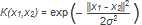### Recognition methods in image processing

www.spiroprojects.com
Image recognition is the process of identifying and detecting an object or a feature in a digital image or video. This concept is used in many applications like systems for factory automation, toll booth monitoring, and security surveillance. Typical image recognition algorithms include:
• Optical character recognition
• Face recognition
• Scene change detection
Specific image recognition applications include classifying digits using HOG features and an SVM classifier
(Figure 1).
A support vector machine (SVM) is a supervised learning algorithm that can be used for binary classification or regression. Support vector machines are popular in applications such as natural language processing, speech and image recognition, and computer vision.
A support vector machine constructs an optimal hyperplane as a decision surface such that the margin of separation between the two classes in the data is maximized. Support vectors refer to a small subset of the training observations that are used as support for the optimal location of the decision surface.
Support vector machines fall under a class of machine learning algorithms called kernel methods and are also referred to as kernel machines.
Training for a support vector machine has two phases:
1. Transform predictors (input data) to a high-dimensional feature space. It is sufficient to just specify the kernel for this step and the data is never explicitly transformed to the feature space. This process is commonly known as the kernel trick.
2. Solve a quadratic optimization problem to fit an optimal hyperplane to classify the transformed features into two classes. The number of transformed features is determined by the number of support vectors.
Only the support vectors chosen from the training data are required to construct the decision surface. Once trained, the rest of the training data are irrelevant.
Popular kernels used with SVMs include:
Type of SVM Mercer Kernel Description
Gaussian or Radial Basis Function (RBF)One class learning. σ is the width of the kernel
Linear K(x1,x2) = x1Tx2 Two class learning.
Polynomial K(x1,x2) = (x1Tx2 + 1)p p is the order of the polynomial
Sigmoid K(x1,x2) = tanh (β0 x1Tx2 + β1) It is a mercer kernel for certain  β0 and β1 values only

Figure 1. Digit classification using Histogram of Oriented Gradients (HOG) feature extraction of image (top) and SVMs. See this example for source code and explanation.
Cross correlation can be used for pattern matching and target tracking as shown in Figure 2.

Figure 2. Using normalized cross-correlation to recognize specific chips on a circuit board. See example for details.
An effective approach for image recognition includes using a technical computing environment for data analysis, visualization, and algorithm development.

Previous
Next Post »